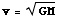Feeds:
Posts

## Dark Matter

Whenever I see a new article about dark matter appear online there seems to be a mandatory accompaniment of comments revolving around the feasibility of something we cannot see. Here is a basic run through of how we know dark matter is out there without the math, mostly.

The Theory:

It starts with assuming simple circular motion of stars in a spiral galaxy. Here gravity is holding the stars in the motion, so the gravitation acceleration is equal to the centripetal acceleration:Where m is the mass of the star, M is the mass of everything within the stars orbit, v is the tangential velocity of the star, r is the distance from the center of the galaxy and G is the gravitational constant. Rearranging this we arrive at:This may lead us to think that the velocity is constant the farther out we go in the spiral galaxy, except that M depends on the radial distance r. The dependency of mass on the radial distance is not an easy equation (meaning of course that I could not do it from first principles on the back of a notesheet). In the end the theoretical plot of galactic velocity versus radius is shown towards the end of this post as line A.

The Evidence:

Alright so now we have this sweet theory of how the galactic velocities should look. I mean we know it is a good thing since we also have a graph, and graphs are never a bad thing. With theory in hand we trot off to our nearest observatory to do some spectral line analysis of a spiral galaxy to determine the red shift along the radial axis. Ah good times.

So what does that mean? Well stuff that moves away from us lengthens the wavelength of light emitted so it appears red. Stuff moving towards us is slightly bluer. Kind of like the Doppler effect when a siren zooms by, the pitch goes up (shorter wavelength) as it approaches and depends when it moves away (longer wavelength). Sort of like that, except not really. We look at a spiral galaxy (let us say edge on) and find the red shift all along the diameter, from there we get the velocity (v = c*z, with v as the velocity, c the speed of light and z the redshift). After we account for the part where the entire galaxy is moving away from us we get a nice profile of the galactic velocity as a function of radius. This is curve B in this graph:

See our nice A line from the theory? See this new B line from what we actually see? See the big gap between them? That is where dark matter comes in. The circular velocity is determined by the mass of stuff within the orbit and so there must be more mass then our original theory predicts. Something is there that is massive and dark (since we cannot see it). This dark stuff. With an Astrophysicists naming ability it is dubbed Dark Matter.

One question that seems to come up a lot: what if the theory of gravity is wrong? Well it is actually wrong, that is why Einstein came up with general relativity. If we altered the theory to explain away dark matter then a lot of other things the theories predict, that are right, would now be wrong. We just use Newtons theory of gravity since it is accurate enough is most cases but it is not perfect. The best way I have heard it said, “It is good for ‘stuff falls down'”.

With dark matter we do not know what it is. We do know where it is and roughly how much there is. Now we just need to find out what it is made of.

My guess: clouds of Higgs Bosons floating around in space. I am sure that is it.